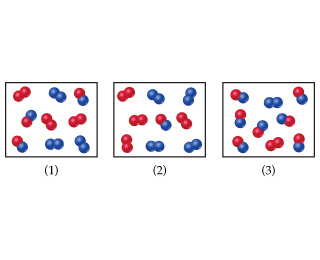# Problem: Consider a reaction A2(g) + B2(g) ⇌ 2AB(g), with atoms of A shown in red here and atoms of B shown in blue. If Kc = 1, which system is at equilibrium?

###### FREE Expert Solution

We are being asked to identify if the system is in equilibrium.

We will use the reaction quotient, Q, to determine if the chemical reaction would be at equilibrium or not.

The formula for Q is:

Depending on if Q is greater than or less than K, our reaction will shift to attain equilibrium by reaching the equilibrium constant K:

81% (380 ratings)###### Problem Details

Consider a reaction A2(g) + B2(g) ⇌ 2AB(g), with atoms of A shown in red here and atoms of B shown in blue.If Kc = 1, which system is at equilibrium?

Frequently Asked Questions

What scientific concept do you need to know in order to solve this problem?

Our tutors have indicated that to solve this problem you will need to apply the The Reaction Quotient concept. You can view video lessons to learn The Reaction Quotient. Or if you need more The Reaction Quotient practice, you can also practice The Reaction Quotient practice problems.

What professor is this problem relevant for?

Based on our data, we think this problem is relevant for Professor Moloney's class at ODU.